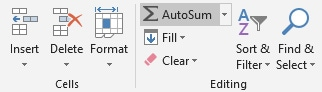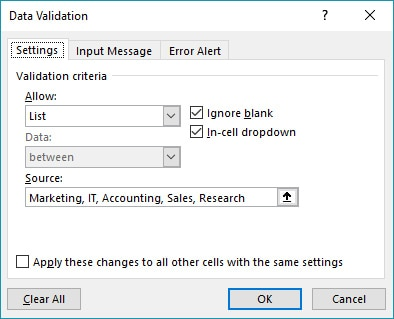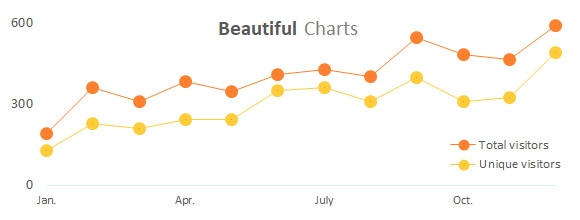# How to use SUM, SUMSQ, SUMIF and SUMIFS functions in Excel

Understanding how to use SUMIF and SUMIFS functions in Excel is easier than you think. However, in order to properly use each of these functions you first need to learn what are the parameters used for computation and the expected end result.

After reading this article you will be able to use each of the functions with ease.

## How to use SUM and SUMSQ functions

SUM and SUMSQ are some of the most basic Excel functions. They are easy to use and there are no specific parameters or conditions required.

SUM function adds up all the numbers and returns the total amount, while SUMSQ function takes the square value of each number and returns the total amount resulted from adding all the values. The syntax is the same for both functions.

Syntax:
`=SUM(number1,[number2],...)`
`=SUMSQ(number1,[number2],...)`

You can use numbers (like 5 or 11), cells (D1, F3, and so on), ranges or even cell or range names. Please keep in mind that you are limited to a maximum of 255 numbers.

Now let’s dive in the actual formula and look at some examples of how to use those functions.

### Examples using SUM and SUMSQ functions

Example 1
Let’s assume we need to calculate the salaries of all employees. We can easily achieve this by using the SUM function:
`=SUM(G2:G25)`
To select the range you just need to select G2 and with your left mouse click pressed drag all the way down to G25. Then close the brackets (parenthesis) and hit Enter.

Example 2:
You can also name a range of cells and use that name in the SUM function. For this example we have named G2:G25 as “Salaries” and used the formula =SUM(Salaries) to calculate the total annual salaries. The result is the same as in Example 1, the only difference is the method used for achieving this.

Example 3
Additionally, in our file we also calculated the total annual salaries for employees working in IT. For this we used the following code:
`=(G3,G7,G15:G17,G21,G24)`
In this case, instead of a single range we just picked the cells we were interested in and skipped the rest of the divisions. As you can see you can combine cells with ranges in the same SUM formula.

However, this method is prone to human errors since it requires you to manually select the salaries of employees working in IT. This means that you can easily select a wrong cell or miss one that should have been included. This is when more advanced and versatile functions like SUMIF or SUMPRODUCT come into play.

Example 4
Another quick way to sum all the cells from a table is to use the Autosum option from the Excel menu. For this, all you need to do is select the cell underneath the last value on the column and press Autosum. You can also make a selection of the cells you want to add up and press the button. The Autosum option is available in the Home tab of the Ribbon, in the Editing section.Keep in mind that this only works with continuous ranges. This means that if you have an empty cell on your column Excel will only take into account the last range starting from the empty cell and ending at the bottom of the column. If you want to add up all the values from a column you cannot use the Autosum option since it will not return the correct value.

Example 5
Using SUMSQ we can easily calculate the square values from cells or range and sum all the values. In this example we have taken cells L9, L10, L11 and L12 and used the formula `=SUMSQ(L9:L12)`. This can be translated into 2^2 + 3^2 + 4^2 + 5^2 = 4 + 9 + 16 + 25 = 54. Just like the SUM function you can use values, cells, arrays or a mix of those. For example, you can type `=SUMSQ(2,3,A8,C11:C20)` and the function will still work correctly.

## How to use SUMIF and SUMIFS functions

SUMIF and SUMIFS functions add more functionalities to the basic SUM formula by introducing selection criteria. This means that you can take a range and only add up certain cells, while completely ignoring the rest. The difference between the two functions is given by the number of criteria used. SUMIF searches the range using only one criteria, while SUMIFS can be used with as much as 127 criteria/conditions pairs.

The syntax for the two functions is explained below:
`=SUMIF(range,criteria,sum_range)`
`=SUMIFS(sum_range, range1, criteria1, [range2], [criteria2], ...)`

Please note the difference in SUMIF syntax. Since it only has one condition, the syntax is changed and the sum_range parameter has been moved to the end. This means that you first have to set the search criteria and only afterwards define the range where it should be applied.

Parameters:
sum_range – The range containing the values to be summed.
range1 – The range to compare against the first criteria.
criteria1 – The criteria to use on range1.
range2 – [optional] The range to compare against the second criteria.
criteria2 – [optional] The criteria to use on range2.

Note: Please keep in mind that each additional range used needs to have the same number of rows and columns. Otherwise, the functions will return an error.

SUMIF and SUMIFS function support logical operators (>,<,<>,=) and wildcards (*,?) for partial matching.

Please note that the following named ranges have been used:
`Division: range (E2:E25)`
`Seniority: range (F2:F25)`
`Salaries: range (G2:G25)`
`Surname: range (C2:C25)`

Example 1
Once we have all our ranges ready we can use the SUMIF formula to quickly calculate the annual salaries for the employees working in IT department. The formula can be written as
`=SUMIF(Division,"IT",Salaries)`
or if you prefer to use the cell reference
`=SUMIF(E2:E25,"IT",G2:G25)`

Example 2
Using the SUMIFS formula we can add more criteria based on our needs. Let’s say we need to sum all the salaries for IT staff with a Mid level of seniority. We can obtained this using
`=SUMIFS(Salaries;Division;"IT";Seniority;"Mid level")`
or
`=SUMIFS(G2:G25;E2:E25;"IT";F2:F25;"Mid level")`

Example 3
Furthermore, you can customize the input values for the criteria using Data Validation. This will allow you to use a predefined drop-down list in order to select which criteria you want to apply to your SUMIF or SUMIFS function. In cells J22 and J23 I have created a predefined list for the Division and Seniority level. By changing the values you can easily recalculate the total salaries for all the possible combinations.

Creating a predefined list can be easily achieved using Data Validation. For this, all you need to do is go to the Data tab on the Excel Ribbon, and select Data Validation in the Data Tools section.On the pop-up window select the Settings tab and pick List as the Validation criteria. In the source field you can insert your values separated by comma, select a cell range to create the list or insert the name of a predefined range containing the values you want to add you your list. Since we only have a handful of options I have pasted them as comma separated values and clicked OK.You can customize the Input Message and Error Alert. These are optional but using them will help the users to better understand the purpose of your drop-down list and the error message in case they insert an invalid name.

Example 4
As I mentioned before you can also use wildcards (*,?) for partial matching. Using the SUMIF function in cell M2 I have calculated the salaries of all employees with surnames starting with letter “T”. I know this would be irrelevant in a real life scenario, but it can be used for the purpose of understanding how wildcards can be applied to a criteria. We can write the formula as
`=SUMIF(Surname,"T*",Salaries)`
or
`=SUMIF(C2:C25,"T*",G2:G25)`

I hope that after reading this article you are able to to better understand how those functions work. In case you need more details or have questions on how to use them in your projects, please leave a comment and I will get back to you as soon as possible.

If you have additional questions please let me know by posting a comment.

#### Add comment

This site uses Akismet to reduce spam. Learn how your comment data is processed.

#### Topics## Improve your Excel skills

Learn how to work with functions, create complex formulas and design beautiful charts. All free.